## learn javascript

Learn JavaScript,  is a beginner’s course that gives you a way to code through fun and interactive tutorials, in a relaxed manner.

You will learn all the basics of using the computer and the internet as you work through many different coding challenges.

I intend to make this course user friendly so that no matter what your skill level is, it should be easy to follow and start programming right now in JavaScript!

### What is JavaScript?

It is a computer programming language, we use it to modify the website content. It can calculate, validate and manipulate the data.

We write JavaScript code on the HTML page.

JavaScript is a language you must learn to use. If you already know computer programming then it will be easy to understand it.

There is a difference between Java and Javascript. they are totally different.

JavaScript helps you to change the layout, modify the content and decorate the website.

You can perform mathematics functions and solve the equations by using JavaScript on a website.

### What is Internal, External and Inline JavaScript?

In Internal JavaScript we can write JavaScript codding between <script> </script> tags.

While for External JavaScript you have to create an external file with the extension of “.js” (filename.js). That we attach it with the HTML document.

We can use Inline JavaScript the same as we do in CSS. Same three methods to use JavaScript as CSS.

### How to write JavaScript?

As we know JavaScript codes we type in <script> </script>

<script type = “text/javascript”>

We use document.write command in JavaScript to display output in a web browser.

### How to write the first JavaScript Code Example?

Open notepad and type the following JavaScript code.

<html>
<title>
My first JavaScript Page
</title>
<body>
<script type = “text/javascript”>
document.write(“My first JavaScript Page”);
</script></body></html>

Save this “filename.html” for example “myjsfile.html” you can use any file name but add “.html” after the file name.

Output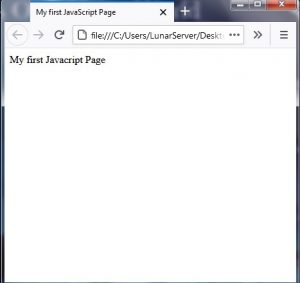### How to add  Comments in JavaScript?

There are two types of comments we can add to our JavaScript codding.

Comments are not visible on the output screen.  Web developer types the comments for understanding the codes types and uses.

For single-line comment

For multi-line comment

This is Lunar Computer College *\

### Enable Disable JavaScript in a Google Chrome and Firefox

In Google Chrome Click on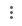the top right corner of Chrome under the close icon.

Then click on Settings On the next page scroll down to last and click on Advanced now under the Privacy and security click on Content settings.

Here you can find Javascript (Allowed/Blocked). You can change the setting by clicking on (Allowed or blocked)

### Enable disable JavaScript in Firefox

Open firefox type about:config in the address bar of Firefox and press Enter.

on the next window type javascript in the search bar and press Enter.

now you can see javascript in the list that you can enable and disable by double click on it.

### What is noscript tag in JavaScript?

Use this tag after the end of </script> in the html. The purpose of using this code is to display a warning message if javascript would be disabled or if the web browser you are using is old.

### How to use variables in JavaScript?

In JavaScript, we can use variables to solve the math equations or to store the string or numeric values.

The variable contains alphabets, numbers, dollar sign (\$) and underscore (_).

The variable name must be started from the alphabets.

A variable name is case sensitive (n=10 and N = 100 are the different variables in JavaScript)

\$ and _ can be used at the start of a variable

Reseved words like javascript or script can not be used as the variable name.

In javascript we use var to assign the  value in variable

var x = 9

x is a variable and 9 is the value.

Two words variable in javascript stName = “Rana”

#### How to add values in JavaScript using variables?

Open notepad and type the following codes to add the numeric values in javascript.

<!DOCTYPE html>
<html>
<title>
Adding numeric values using variables
</title>
<body>
<script type = “text/javascript”>
var x = 5
var y = 10
var z = x+y
document.write(x);
document.write(“+”);
document.write(y);
document.write(“=”);
document.write(z);
</script>
</body>
</html>

Save this with a .html extension like addme.html and open it on the web browser.

Output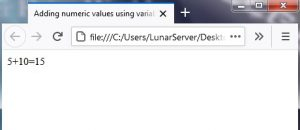#### How to Add Subtract Multiply Divide in Javascript?

We can add subtract multiply and divide the numeric values the same as we add the numeric values in the upper example.

10 + 5 = 15
10 – 5 = 5
10 * 5 = 50
10 / 5 = 2

you need to use a document.write(“</br>”); tag to get the output in different lines otherwise your output will be like this

10 + 5 = 1510 – 5 = 510 * 5 = 5010 / 5 = 2

### How to get input from user in JavaScript?

var n\$ = prompt (“your message on screen for string values”);

var n = prompt (“your message on screen for numeric values”);

### For Loop in JavaScript

for (var n =1;n<=10;n++)

<html>
<title>
My first 10 Natural Number with for loop in JavaScript
</title>
<body>
<script type = “text/javascript”>

for (var k =1;k<=10;k++)

{

document.write(k);

document.write(“</br>”);

}
</script></body></html>

Output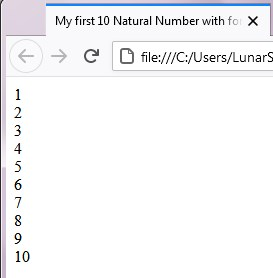### How to print a table of any given number in JavaScript?

<!DOCTYPE html>
<html>
<title>
Number Table Software
</title>
<script type = “text/javascript”>
var table = prompt(“Which Table do you want to learn”,””);
document.write(“Table of ” + table);
document.write(“<div>”);
document.write(“===============”);
for(var i=1;i<=10;i++)
{
document.write(“<div>”);
document.write(table + ” x ” + i + ” = ” + i*table);
document.write(“</div>”);
}
document.write(“===============”);
</script>
</html>

Save the upper codding in the table.html om notepad

OutPut

When you run this HTML file on a web browser, the following windows appear asking you which table you want to learn.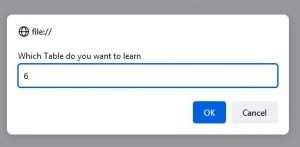As I typed 6 in this dialogue windows.

So you can see the computer will display the table of 6 as shown in the image below.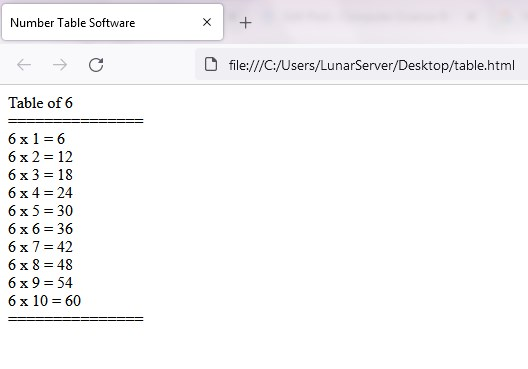### Make a number table software in javascript with start and end value given by user

<!DOCTYPE html>
<html>
<title>
Number Table Software
</title>
<script type = “text/javascript”>
var table = prompt(“Which Table do you want to learn”,””);
var stable = prompt(“Where do you want to start Table”,””);
var etable = prompt(“Where do you want to end the Table”,””);
document.write(“Table of ” + table);
document.write(“<div>”);
document.write(“===============”);
for(var i=stable;i<=etable;i++)
{
document.write(“<div>”);
document.write(table + ” x ” + i + ” = ” + i*table);
document.write(“</div>”);
}
document.write(“===============”);
</script>

SiteMap